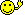CodeGuru Home VC++ / MFC / C++ .NET / C# Visual Basic VB Forums Developer.com

1.Junior MemberJoin Date
Apr 2013
Posts
1

##Xcel Sheet Insert

I'm new to these forums and to coding so please don't eat me alive if I come across as a complete amateurI want to pull information from my computer and then add this information to the Excel Sheet. How do I do this? I have a column in B in Excel Sheet as that's were I'd like the information to go. Here is my code:

Code:
```Option Explicit

'declare variables
Dim objDomain, objFSO, objExcel, objSheet, objWMI, objItem
Dim userSheet, intRow, colItem
Dim strComputer, strIP

userSheet = "c:\Computers.xlsx"

Set objExcel = Createobject("Excel.Application")

'creating a connection to file system object
Set objFSO = CreateObject("Scripting.FileSystemObject")

Set objSheet = objExcel.Workbooks.Open(userSheet)
intRow = 3

'loop that gets data from cells
Do Until objExcel.Cells(introw,1).Value = ""
strIP = Trim(objExcel.Cells(introw, 1).Value)

strComputer = strIP

WScript.Echo(strComputer)

Set objWMI = GetObject("winmgmts:\\" & strComputer & "\root\cimv2")

Set colItem = objWMI.ExecQuery("Select * from Win32_OperatingSystem",,48)
For Each objItem In colItem
WScript.Echo "Name " & objItem.Name
Next

intRow = intRow + 1

Loop

WScript.Quit```
If I need to provide anything more or something is unclear please let me know. I'd let to get this resolved as quick as possible as time is pressing for this.Reply With Quote

2. ## Re: Xcel Sheet Insert

I'm assuming that column A contains the names of the computers you want to query, and that the first computer listed is on (int)row 3. I am also assuming that you want to determine the o/s of each one. Writing the data back is just a minor variation on the line where you read the value (in your code
Code:
`strIP = Trim(objExcel.Cells(introw, 1).Value)`
- just set the value instead. What you should be doing though is initialising the Excel object, then creating a workbook object from that which references the spreadsheet file, then a worksheet object (which will allow you to choose sheets other than Sheet1 for example). The other thing your script needs is error checking as the WMI call might fail if the computer is switched off.
This script should do what you want. It also attempts to just read the o/s name rather than the active partition where it is:

Code:
```Option Explicit
'declare variables
Dim objDomain, objExcel, objSheet, objWMI, objItem
Dim userSheet, intRow, colItem, objWorkbook
Dim strComputer, strOS
userSheet = "c:\Scripts\Computers.xlsx"
Set objExcel = Createobject("Excel.Application")
objExcel.Visible = False
'creating a connection to file system object
Set objWorkbook = objExcel.Workbooks.Open(userSheet)
Set objSheet = objWorkbook.Worksheets(1)
intRow = 3
'loop that gets data from cells
On Error Resume Next
Do Until objSheet.Cells(introw,1).Value = ""
strComputer = Trim(objSheet.Cells(introw, 1).Value)
Set objWMI = GetObject("winmgmts:\\" & strComputer & "\root\cimv2")
If Err.Number <> 0 Then
strOS = "***NOT AVAILABLE"
Err.Clear
Else
Set colItem = objWMI.ExecQuery("Select * from Win32_OperatingSystem",,48)
For Each objItem In colItem
If InStr(objItem.Name,"|") Then
strOS = Split(objItem.Name,"|")(0)
Else
strOS = objItem.Name
End If
Next
End If
objSheet.Cells(introw, 2).Value = strOS
WScript.Echo strComputer & VBTab & strOS
intRow = intRow + 1
Loop
objWorkbook.Save()
objExcel.Quit```Reply With Quote

excel, help wanted, insert, vbscript####Posting Permissions

• You may not post new threads
• You may not post replies
• You may not post attachments
• You may not edit your posts
•